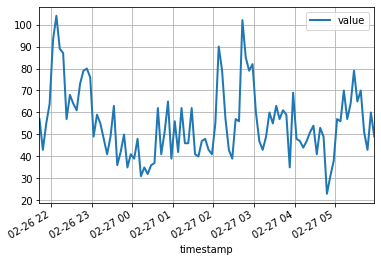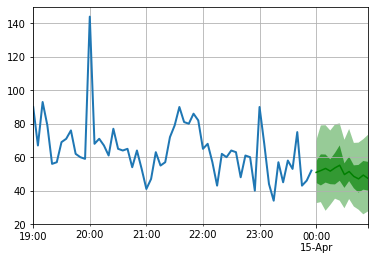# Pytorch Ts

PyTorch based Probabilistic Time Series forecasting framework based on GluonTS backend
Alternatives To Pytorch Ts
Darts5,956710 hours ago25June 22, 2022234apache-2.0Python
A python library for user-friendly forecasting and anomaly detection on time series.
Tsai3,495112 days ago41April 19, 202232apache-2.0Jupyter Notebook
Time series Timeseries Deep Learning Machine Learning Pytorch fastai | State-of-the-art Deep Learning library for Time Series and Sequences in Pytorch / fastai
Pytorch Forecasting2,858410 days ago33May 23, 2022391mitPython
Time series forecasting with PyTorch
Deep Learning Time Series1,811
9 months ago8apache-2.0Jupyter Notebook
List of papers, code and experiments using deep learning for time series forecasting
Awesome_time_series_in_python1,811
4 months ago4
This curated list contains python packages for time series analysis
Flow Forecast1,467
a day ago94gpl-3.0Python
Deep learning PyTorch library for time series forecasting, classification, and anomaly detection (originally for flood forecasting).
Pmdarima1,36464512 days ago21February 22, 202242mitPython
A statistical library designed to fill the void in Python's time series analysis capabilities, including the equivalent of R's auto.arima function.
Pytorch Ts1,000
a day ago9April 24, 202262mitPython
PyTorch based Probabilistic Time Series forecasting framework based on GluonTS backend
Autots74718 days ago40June 20, 20227mitPython
Automated Time Series Forecasting
N Beats686
3 months agomitPython
Keras/Pytorch implementation of N-BEATS: Neural basis expansion analysis for interpretable time series forecasting.
Alternatives To Pytorch Ts
Select To Compare

Alternative Project Comparisons

# PyTorchTS

PyTorchTS is a PyTorch Probabilistic Time Series forecasting framework which provides state of the art PyTorch time series models by utilizing GluonTS as its back-end API and for loading, transforming and back-testing time series data sets.

## Installation

``````\$ pip3 install pytorchts
``````

## Quick start

Here we highlight the the API changes via the GluonTS README.

``````import matplotlib.pyplot as plt
import pandas as pd
import torch

from gluonts.dataset.common import ListDataset
from gluonts.dataset.util import to_pandas

from pts.model.deepar import DeepAREstimator
from pts import Trainer
``````

This simple example illustrates how to train a model on some data, and then use it to make predictions. As a first step, we need to collect some data: in this example we will use the volume of tweets mentioning the AMZN ticker symbol.

``````url = "https://raw.githubusercontent.com/numenta/NAB/master/data/realTweets/Twitter_volume_AMZN.csv"
``````

The first 100 data points look like follows:

``````df[:100].plot(linewidth=2)
plt.grid(which='both')
plt.show()
``````We can now prepare a training dataset for our model to train on. Datasets are essentially iterable collections of dictionaries: each dictionary represents a time series with possibly associated features. For this example, we only have one entry, specified by the `"start"` field which is the timestamp of the first data point, and the `"target"` field containing time series data. For training, we will use data up to midnight on April 5th, 2015.

``````training_data = ListDataset(
[{"start": df.index, "target": df.value[:"2015-04-05 00:00:00"]}],
freq = "5min"
)
``````

A forecasting model is a predictor object. One way of obtaining predictors is by training a correspondent estimator. Instantiating an estimator requires specifying the frequency of the time series that it will handle, as well as the number of time steps to predict. In our example we're using 5 minutes data, so `req="5min"`, and we will train a model to predict the next hour, so `prediction_length=12`. The input to the model will be a vector of size `input_size=43` at each time point. We also specify some minimal training options in particular training on a `device` for `epoch=10`.

``````device = torch.device("cuda" if torch.cuda.is_available() else "cpu")

estimator = DeepAREstimator(freq="5min",
prediction_length=12,
input_size=19,
trainer=Trainer(epochs=10,
device=device))
predictor = estimator.train(training_data=training_data, num_workers=4)
``````
``````    45it [00:01, 37.60it/s, avg_epoch_loss=4.64, epoch=0]
48it [00:01, 39.56it/s, avg_epoch_loss=4.2, epoch=1]
45it [00:01, 38.11it/s, avg_epoch_loss=4.1, epoch=2]
43it [00:01, 36.29it/s, avg_epoch_loss=4.05, epoch=3]
44it [00:01, 35.98it/s, avg_epoch_loss=4.03, epoch=4]
48it [00:01, 39.48it/s, avg_epoch_loss=4.01, epoch=5]
48it [00:01, 38.65it/s, avg_epoch_loss=4, epoch=6]
46it [00:01, 37.12it/s, avg_epoch_loss=3.99, epoch=7]
48it [00:01, 38.86it/s, avg_epoch_loss=3.98, epoch=8]
48it [00:01, 39.49it/s, avg_epoch_loss=3.97, epoch=9]
``````

During training, useful information about the progress will be displayed. To get a full overview of the available options, please refer to the source code of `DeepAREstimator` (or other estimators) and `Trainer`.

We're now ready to make predictions: we will forecast the hour following the midnight on April 15th, 2015.

``````test_data = ListDataset(
[{"start": df.index, "target": df.value[:"2015-04-15 00:00:00"]}],
freq = "5min"
)
``````
``````for test_entry, forecast in zip(test_data, predictor.predict(test_data)):
to_pandas(test_entry)[-60:].plot(linewidth=2)
forecast.plot(color='g', prediction_intervals=[50.0, 90.0])
plt.grid(which='both')
``````Note that the forecast is displayed in terms of a probability distribution: the shaded areas represent the 50% and 90% prediction intervals, respectively, centered around the median (dark green line).

## Development

``````pip install -e .
pytest test
``````

## Citing

To cite this repository:

``````@software{pytorchgithub,
author = {Kashif Rasul},
title = {{P}yTorch{TS}},
url = {https://github.com/zalandoresearch/pytorch-ts},
version = {0.6.x},
year = {2021},
}
``````

## Scientific Article

We have implemented the following model using this framework:

``````@INPROCEEDINGS{rasul2020tempflow,
author = {Kashif Rasul and  Abdul-Saboor Sheikh and  Ingmar Schuster and Urs Bergmann and Roland Vollgraf},
title = {{M}ultivariate {P}robabilistic {T}ime {S}eries {F}orecasting via {C}onditioned {N}ormalizing {F}lows},
year = {2021},
url = {https://openreview.net/forum?id=WiGQBFuVRv},
booktitle = {International Conference on Learning Representations 2021},
}
``````
``````@InProceedings{pmlr-v139-rasul21a,
title = 	 {{A}utoregressive {D}enoising {D}iffusion {M}odels for {M}ultivariate {P}robabilistic {T}ime {S}eries {F}orecasting},
author =       {Rasul, Kashif and Seward, Calvin and Schuster, Ingmar and Vollgraf, Roland},
booktitle = 	 {Proceedings of the 38th International Conference on Machine Learning},
pages = 	 {8857--8868},
year = 	 {2021},
editor = 	 {Meila, Marina and Zhang, Tong},
volume = 	 {139},
series = 	 {Proceedings of Machine Learning Research},
month = 	 {18--24 Jul},
publisher =    {PMLR},
pdf = 	 {http://proceedings.mlr.press/v139/rasul21a/rasul21a.pdf},
url = 	 {http://proceedings.mlr.press/v139/rasul21a.html},
}
``````
``````@misc{gouttes2021probabilistic,
title={{P}robabilistic {T}ime {S}eries {F}orecasting with {I}mplicit {Q}uantile {N}etworks},
author={Adèle Gouttes and Kashif Rasul and Mateusz Koren and Johannes Stephan and Tofigh Naghibi},
year={2021},
eprint={2107.03743},
archivePrefix={arXiv},
primaryClass={cs.LG}
}
``````
Popular Forecasting Projects
Popular Series Projects
Popular Machine Learning Categories
Related Searches

Get A Weekly Email With Trending Projects For These Categories
No Spam. Unsubscribe easily at any time.
Python
Pytorch
Time
Series
Time Series
Forecasting